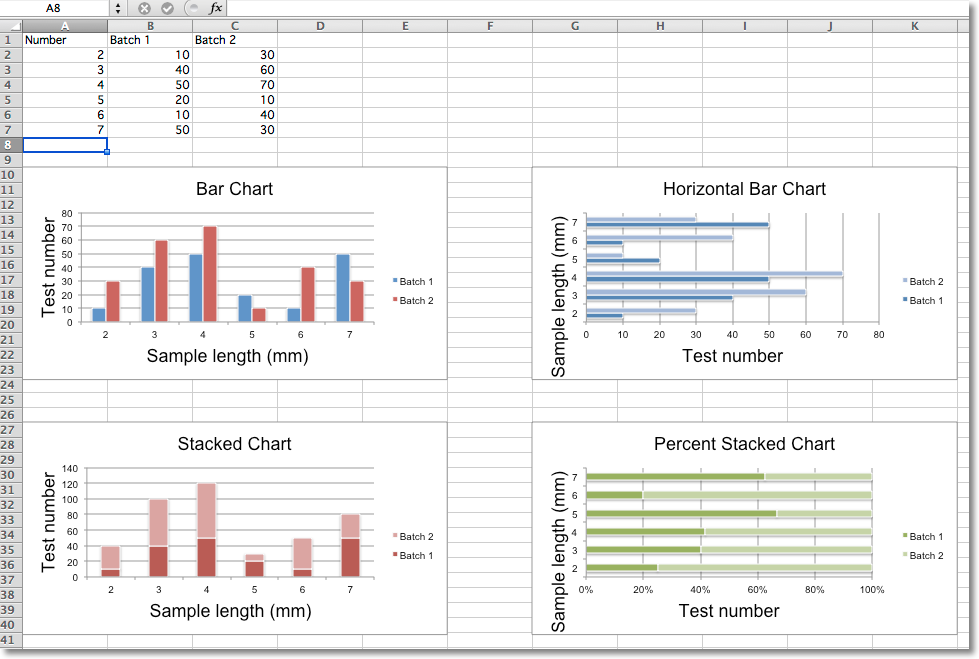# Bar and Column Charts¶

In bar charts values are plotted as either horizontal bars or vertical columns.

## Vertical, Horizontal and Stacked Bar Charts¶

Note

The following settings affect the different chart types.

Switch between vertical and horizontal bar charts by setting type to col or bar respectively.

When using stacked charts the overlap needs to be set to 100.

If bars are horizontal, x and y axes are reversed.```from openpyxl import Workbook
from openpyxl.chart import BarChart, Series, Reference

wb = Workbook(write_only=True)
ws = wb.create_sheet()

rows = [
('Number', 'Batch 1', 'Batch 2'),
(2, 10, 30),
(3, 40, 60),
(4, 50, 70),
(5, 20, 10),
(6, 10, 40),
(7, 50, 30),
]

for row in rows:
ws.append(row)

chart1 = BarChart()
chart1.type = "col"
chart1.style = 10
chart1.title = "Bar Chart"
chart1.y_axis.title = 'Test number'
chart1.x_axis.title = 'Sample length (mm)'

data = Reference(ws, min_col=2, min_row=1, max_row=7, max_col=3)
cats = Reference(ws, min_col=1, min_row=2, max_row=7)
chart1.set_categories(cats)
chart1.shape = 4

from copy import deepcopy

chart2 = deepcopy(chart1)
chart2.style = 11
chart2.type = "bar"
chart2.title = "Horizontal Bar Chart"

chart3 = deepcopy(chart1)
chart3.type = "col"
chart3.style = 12
chart3.grouping = "stacked"
chart3.overlap = 100
chart3.title = 'Stacked Chart'

chart4 = deepcopy(chart1)
chart4.type = "bar"
chart4.style = 13
chart4.grouping = "percentStacked"
chart4.overlap = 100
chart4.title = 'Percent Stacked Chart'

wb.save("bar.xlsx")
```

This will produce four charts illustrating the various possibilities.

## 3D Bar Charts¶

You can also create 3D bar charts

```from openpyxl import Workbook
from openpyxl.chart import (
Reference,
Series,
BarChart3D,
)

wb = Workbook()
ws = wb.active

rows = [
(None, 2013, 2014),
("Apples", 5, 4),
("Oranges", 6, 2),
("Pears", 8, 3)
]

for row in rows:
ws.append(row)

data = Reference(ws, min_col=2, min_row=1, max_col=3, max_row=4)
titles = Reference(ws, min_col=1, min_row=2, max_row=4)
chart = BarChart3D()
chart.title = "3D Bar Chart"